Скачать презентацию Cryptography Public Key Cryptosystems Anita Jones CS 451

2455ae9fc253bd2de073816a7ec19b75.ppt

• Количество слайдов: 38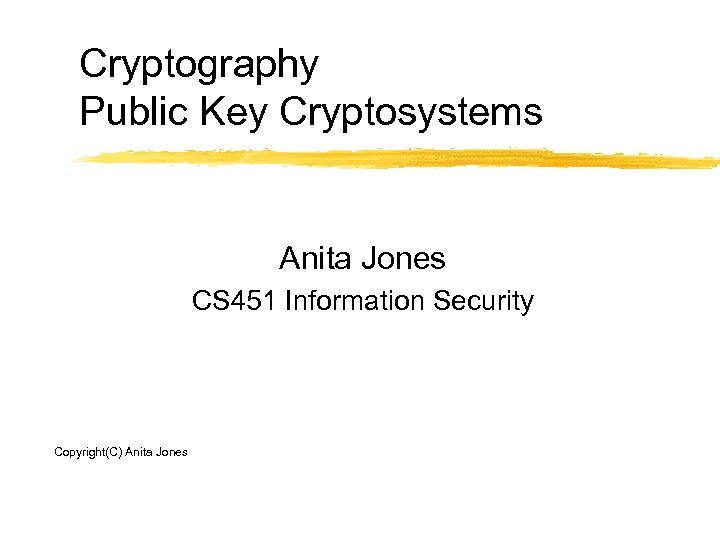Cryptography Public Key Cryptosystems Anita Jones CS 451 Information Security Copyright(C) Anita Jones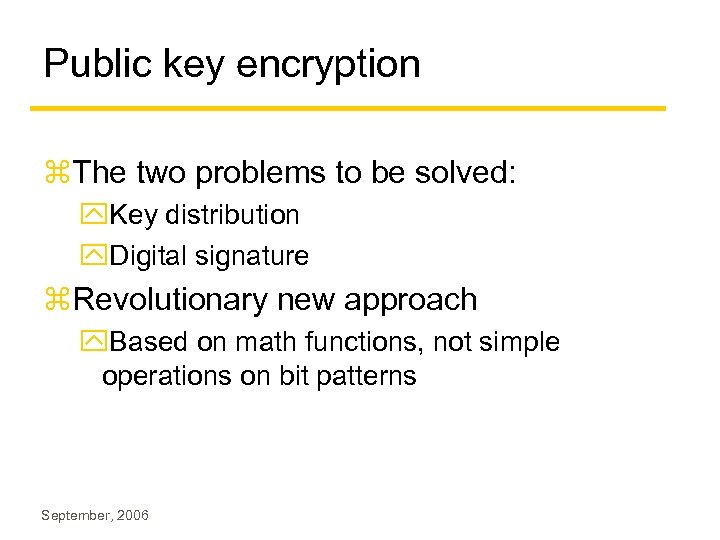Public key encryption z. The two problems to be solved: y. Key distribution y. Digital signature z. Revolutionary new approach y. Based on math functions, not simple operations on bit patterns September, 2006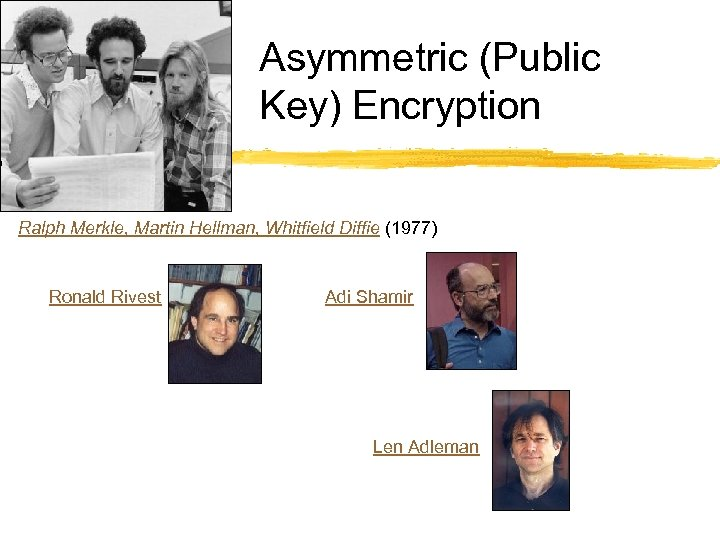Asymmetric (Public Key) Encryption Ralph Merkle, Martin Hellman, Whitfield Diffie (1977) Ronald Rivest Adi Shamir Len Adleman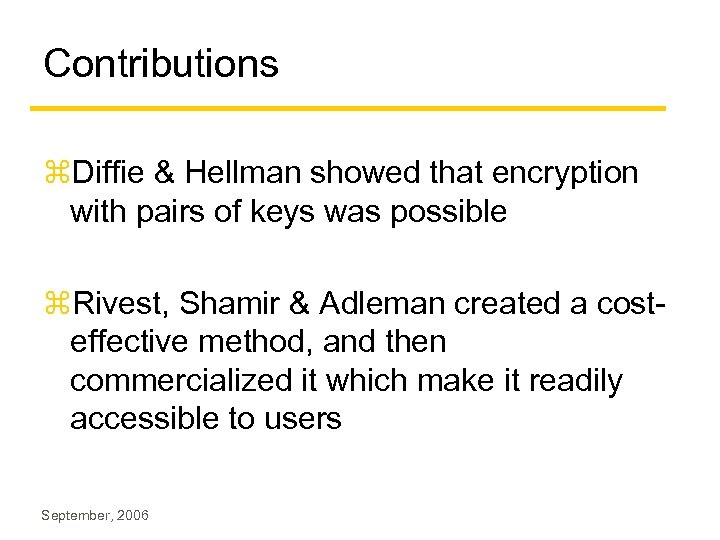Contributions z. Diffie & Hellman showed that encryption with pairs of keys was possible z. Rivest, Shamir & Adleman created a costeffective method, and then commercialized it which make it readily accessible to users September, 2006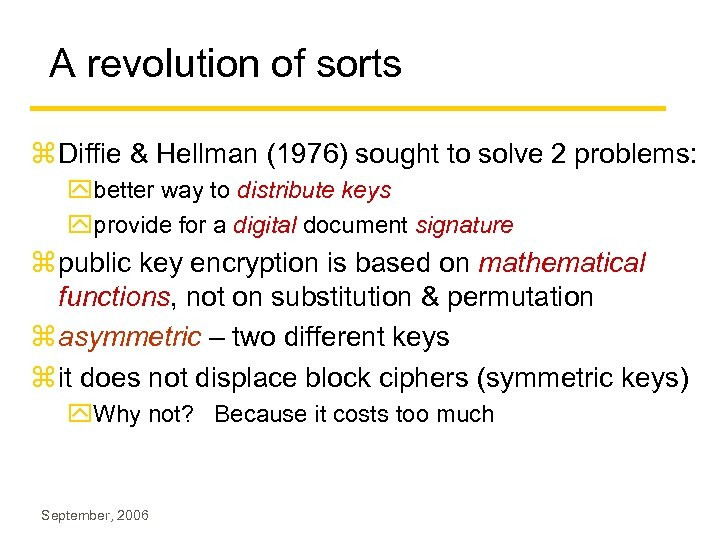A revolution of sorts z Diffie & Hellman (1976) sought to solve 2 problems: ybetter way to distribute keys yprovide for a digital document signature z public key encryption is based on mathematical functions, not on substitution & permutation z asymmetric – two different keys z it does not displace block ciphers (symmetric keys) y. Why not? Because it costs too much September, 2006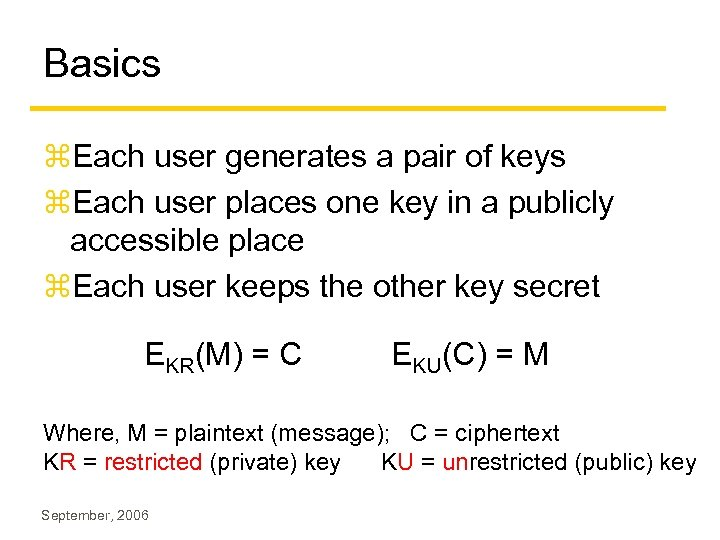Basics z. Each user generates a pair of keys z. Each user places one key in a publicly accessible place z. Each user keeps the other key secret EKR(M) = C EKU(C) = M Where, M = plaintext (message); C = ciphertext KR = restricted (private) key KU = unrestricted (public) key September, 2006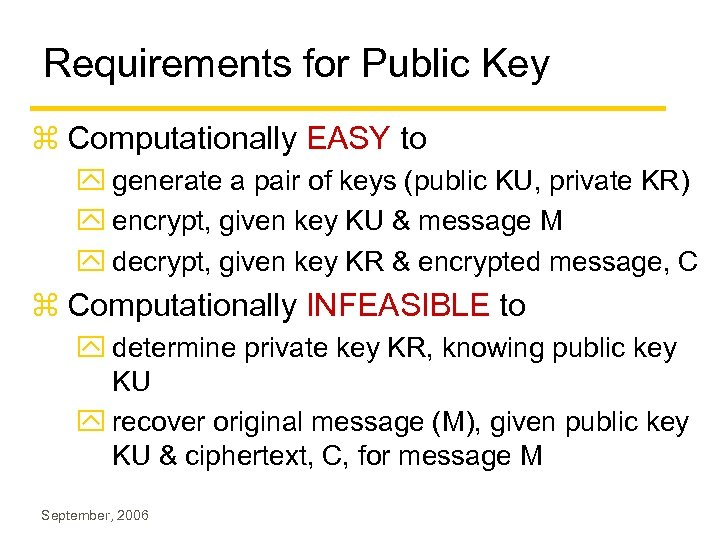Requirements for Public Key z Computationally EASY to y generate a pair of keys (public KU, private KR) y encrypt, given key KU & message M y decrypt, given key KR & encrypted message, C z Computationally INFEASIBLE to y determine private key KR, knowing public key KU y recover original message (M), given public key KU & ciphertext, C, for message M September, 2006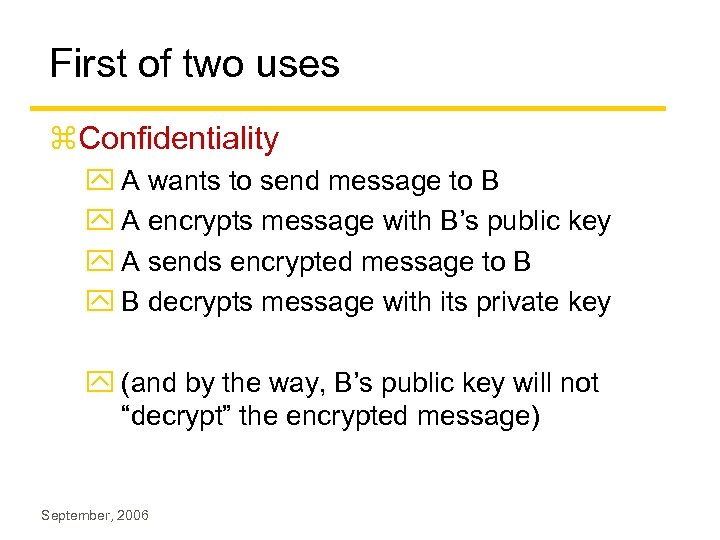First of two uses z. Confidentiality y A wants to send message to B y A encrypts message with B’s public key y A sends encrypted message to B y B decrypts message with its private key y (and by the way, B’s public key will not “decrypt” the encrypted message) September, 2006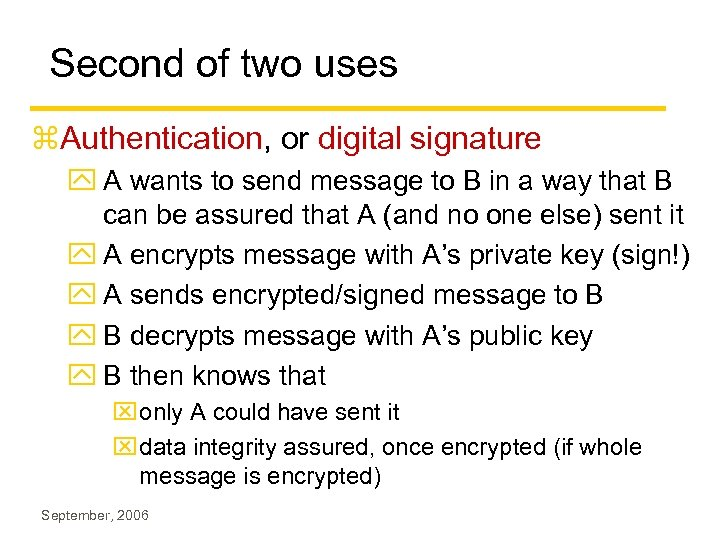Second of two uses z. Authentication, or digital signature y A wants to send message to B in a way that B can be assured that A (and no one else) sent it y A encrypts message with A’s private key (sign!) y A sends encrypted/signed message to B y B decrypts message with A’s public key y B then knows that x only A could have sent it x data integrity assured, once encrypted (if whole message is encrypted) September, 2006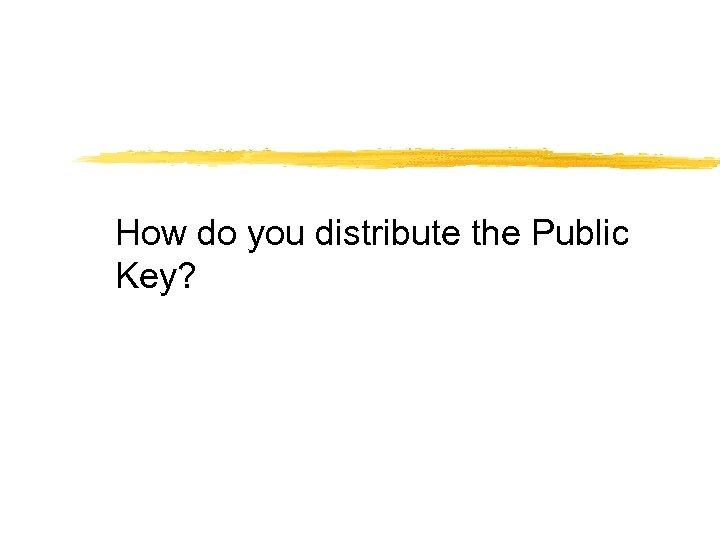How do you distribute the Public Key?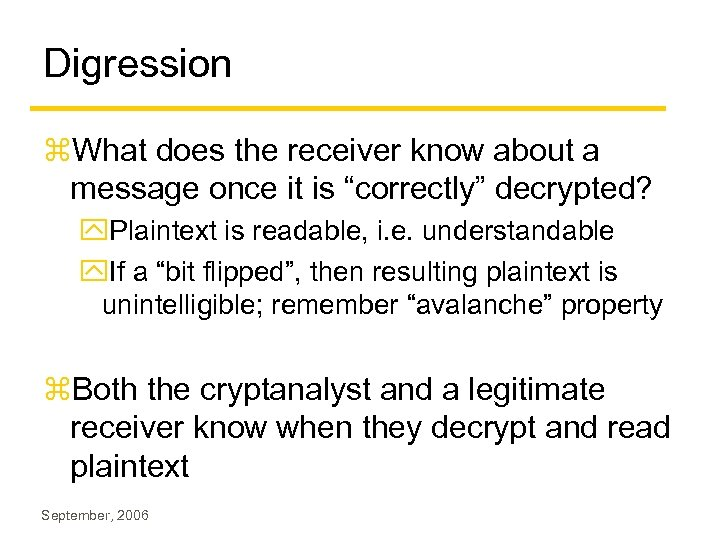Digression z. What does the receiver know about a message once it is “correctly” decrypted? y. Plaintext is readable, i. e. understandable y. If a “bit flipped”, then resulting plaintext is unintelligible; remember “avalanche” property z. Both the cryptanalyst and a legitimate receiver know when they decrypt and read plaintext September, 2006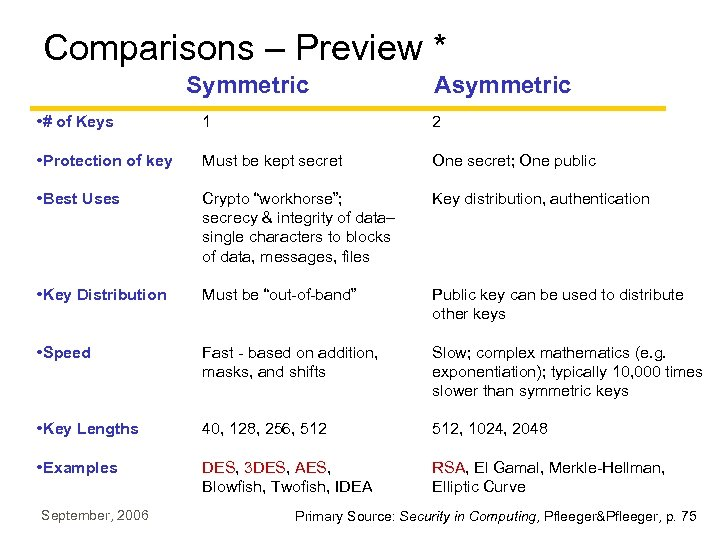Comparisons – Preview * Symmetric Asymmetric • # of Keys 1 2 • Protection of key Must be kept secret One secret; One public • Best Uses Crypto “workhorse”; secrecy & integrity of data– single characters to blocks of data, messages, files Key distribution, authentication • Key Distribution Must be “out-of-band” Public key can be used to distribute other keys • Speed Fast - based on addition, masks, and shifts Slow; complex mathematics (e. g. exponentiation); typically 10, 000 times slower than symmetric keys • Key Lengths 40, 128, 256, 512, 1024, 2048 • Examples DES, 3 DES, AES, Blowfish, Twofish, IDEA RSA, El Gamal, Merkle-Hellman, Elliptic Curve September, 2006 Primary Source: Security in Computing, Pfleeger&Pfleeger, p. 75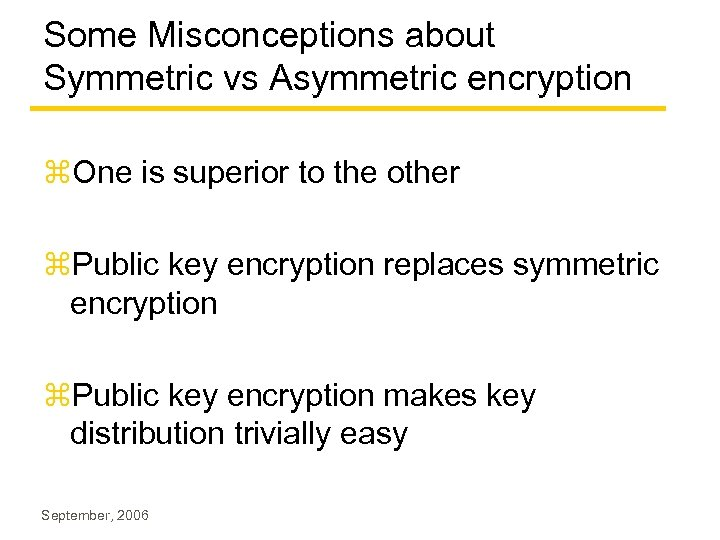Some Misconceptions about Symmetric vs Asymmetric encryption z. One is superior to the other z. Public key encryption replaces symmetric encryption z. Public key encryption makes key distribution trivially easy September, 2006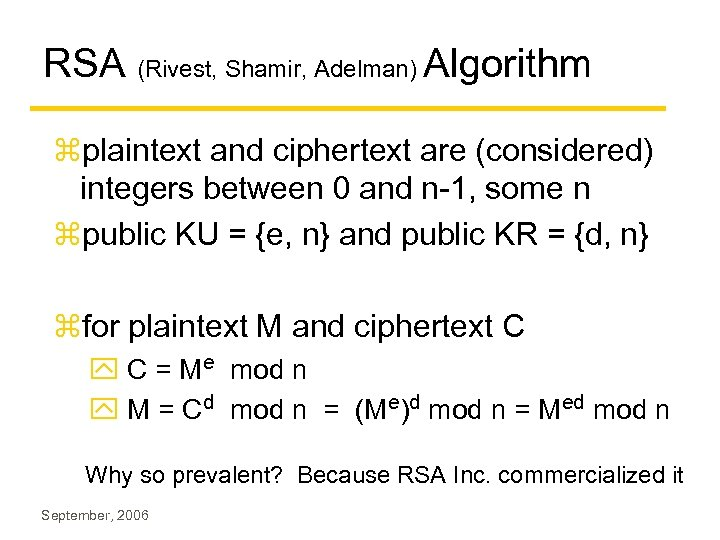RSA (Rivest, Shamir, Adelman) Algorithm zplaintext and ciphertext are (considered) integers between 0 and n-1, some n zpublic KU = {e, n} and public KR = {d, n} zfor plaintext M and ciphertext C y C = Me mod n y M = Cd mod n = (Me)d mod n = Med mod n Why so prevalent? Because RSA Inc. commercialized it September, 2006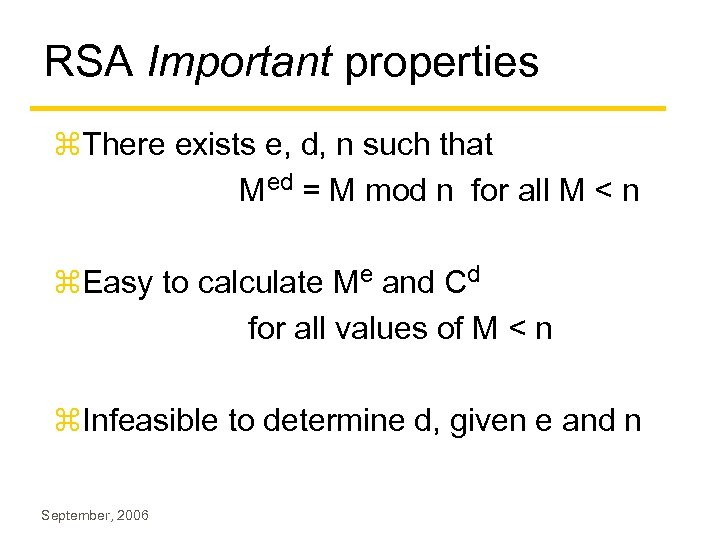RSA Important properties z. There exists e, d, n such that Med = M mod n for all M < n z. Easy to calculate Me and Cd for all values of M < n z. Infeasible to determine d, given e and n September, 2006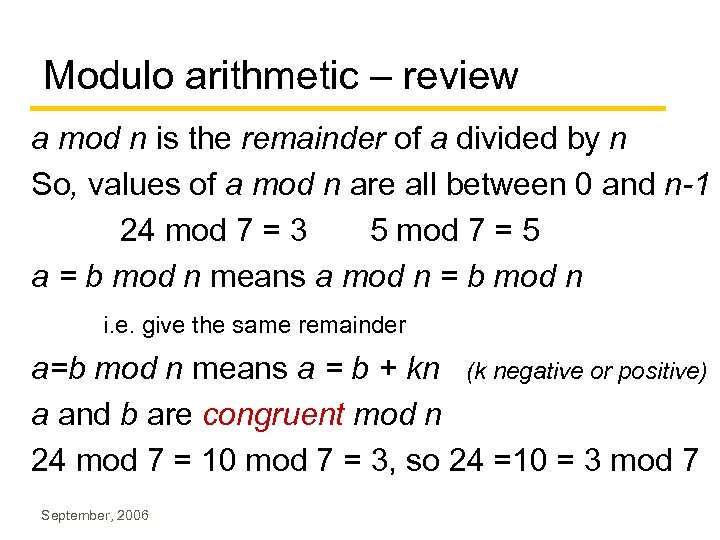Modulo arithmetic – review a mod n is the remainder of a divided by n So, values of a mod n are all between 0 and n-1 24 mod 7 = 3 5 mod 7 = 5 a = b mod n means a mod n = b mod n i. e. give the same remainder a=b mod n means a = b + kn (k negative or positive) a and b are congruent mod n 24 mod 7 = 10 mod 7 = 3, so 24 =10 = 3 mod 7 September, 2006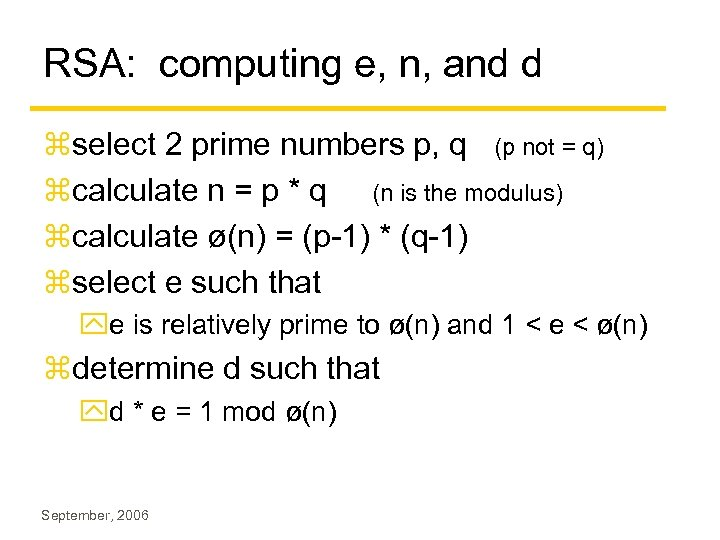RSA: computing e, n, and d zselect 2 prime numbers p, q (p not = q) zcalculate n = p * q (n is the modulus) zcalculate ø(n) = (p-1) * (q-1) zselect e such that ye is relatively prime to ø(n) and 1 < e < ø(n) zdetermine d such that yd * e = 1 mod ø(n) September, 2006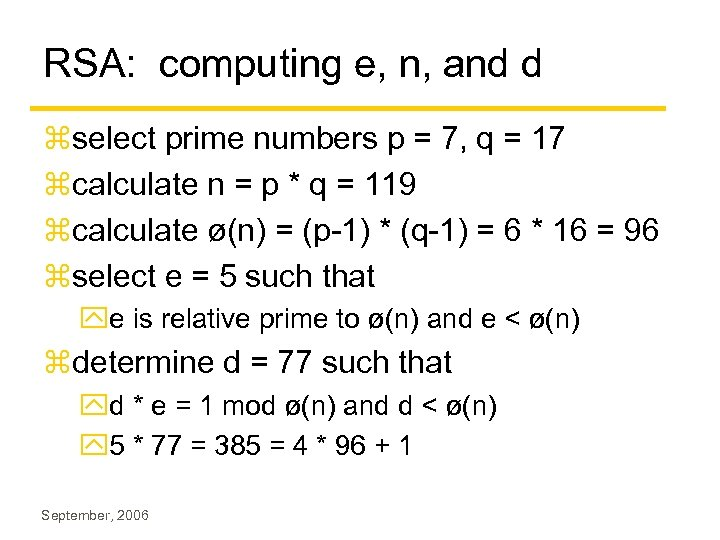RSA: computing e, n, and d zselect prime numbers p = 7, q = 17 zcalculate n = p * q = 119 zcalculate ø(n) = (p-1) * (q-1) = 6 * 16 = 96 zselect e = 5 such that ye is relative prime to ø(n) and e < ø(n) zdetermine d = 77 such that yd * e = 1 mod ø(n) and d < ø(n) y 5 * 77 = 385 = 4 * 96 + 1 September, 2006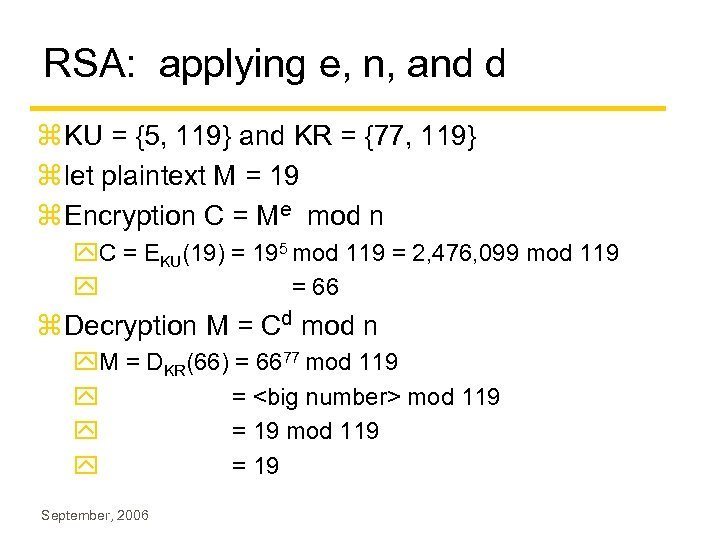RSA: applying e, n, and d z KU = {5, 119} and KR = {77, 119} z let plaintext M = 19 z Encryption C = Me mod n y. C = EKU(19) = 195 mod 119 = 2, 476, 099 mod 119 y = 66 z Decryption M = Cd mod n y. M = DKR(66) = 6677 mod 119 y = mod 119 y = 19 September, 2006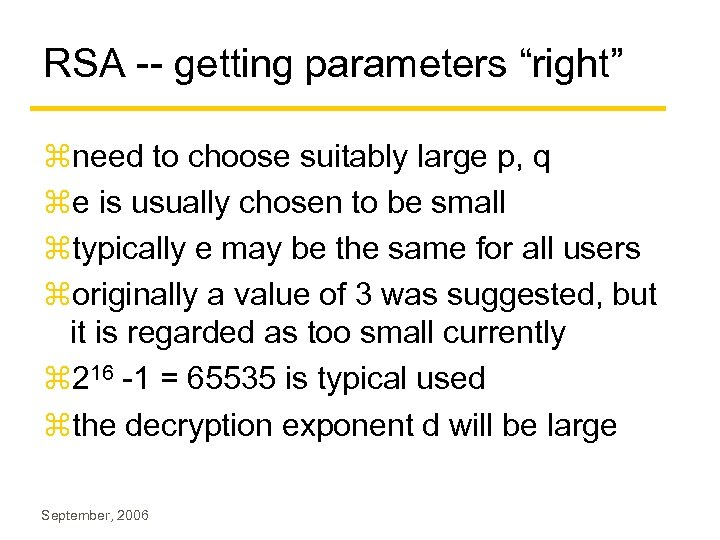RSA -- getting parameters “right” zneed to choose suitably large p, q ze is usually chosen to be small ztypically e may be the same for all users zoriginally a value of 3 was suggested, but it is regarded as too small currently z 216 -1 = 65535 is typical used zthe decryption exponent d will be large September, 2006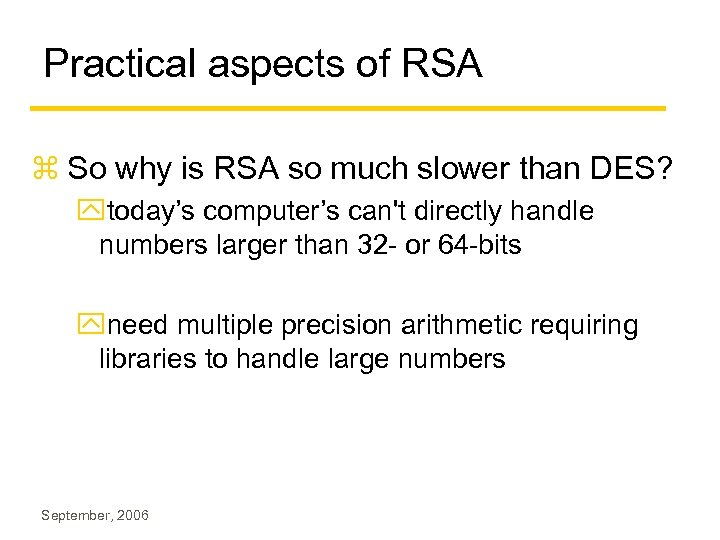Practical aspects of RSA z So why is RSA so much slower than DES? ytoday’s computer’s can't directly handle numbers larger than 32 - or 64 -bits yneed multiple precision arithmetic requiring libraries to handle large numbers September, 2006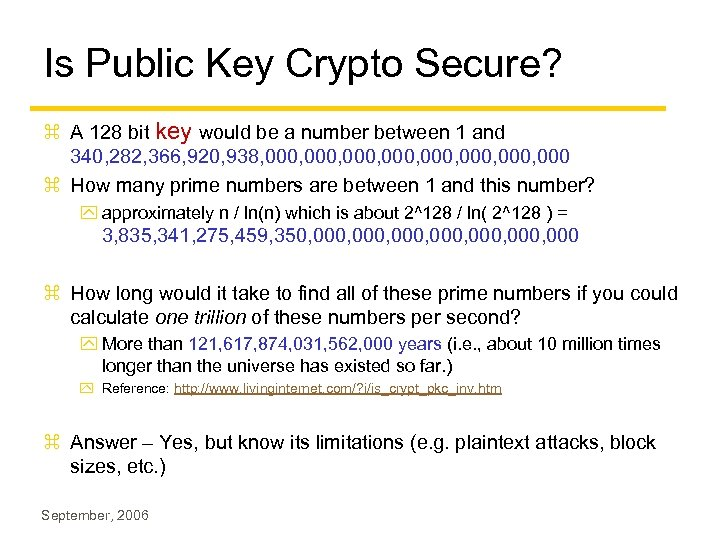Is Public Key Crypto Secure? z A 128 bit key would be a number between 1 and 340, 282, 366, 920, 938, 000, 000, 000 z How many prime numbers are between 1 and this number? y approximately n / ln(n) which is about 2^128 / ln( 2^128 ) = 3, 835, 341, 275, 459, 350, 000, 000, 000 z How long would it take to find all of these prime numbers if you could calculate one trillion of these numbers per second? y More than 121, 617, 874, 031, 562, 000 years (i. e. , about 10 million times longer than the universe has existed so far. ) y Reference: http: //www. livinginternet. com/? i/is_crypt_pkc_inv. htm z Answer – Yes, but know its limitations (e. g. plaintext attacks, block sizes, etc. ) September, 2006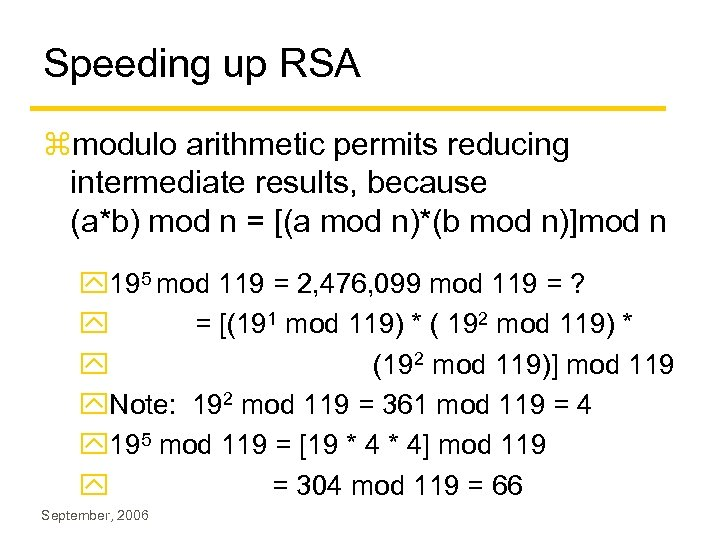Speeding up RSA zmodulo arithmetic permits reducing intermediate results, because (a*b) mod n = [(a mod n)*(b mod n)]mod n y 195 mod 119 = 2, 476, 099 mod 119 = ? y = [(191 mod 119) * ( 192 mod 119) * y (192 mod 119)] mod 119 y. Note: 192 mod 119 = 361 mod 119 = 4 y 195 mod 119 = [19 * 4] mod 119 y = 304 mod 119 = 66 September, 2006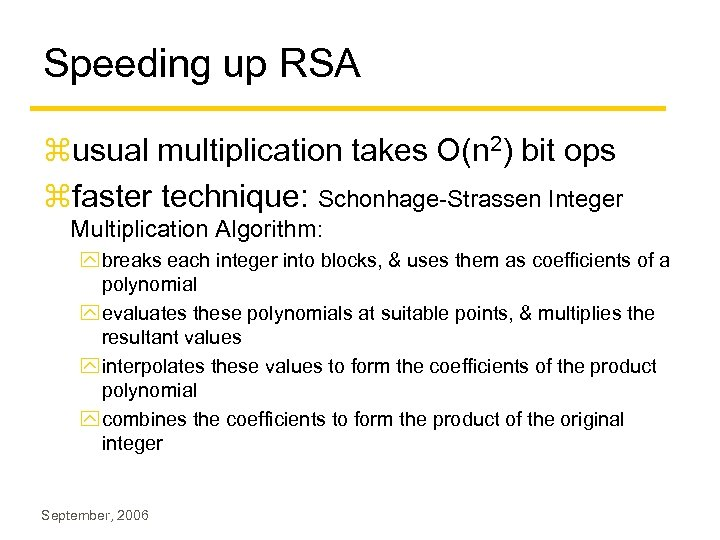Speeding up RSA zusual multiplication takes O(n 2) bit ops zfaster technique: Schonhage-Strassen Integer Multiplication Algorithm: y breaks each integer into blocks, & uses them as coefficients of a polynomial y evaluates these polynomials at suitable points, & multiplies the resultant values y interpolates these values to form the coefficients of the product polynomial y combines the coefficients to form the product of the original integer September, 2006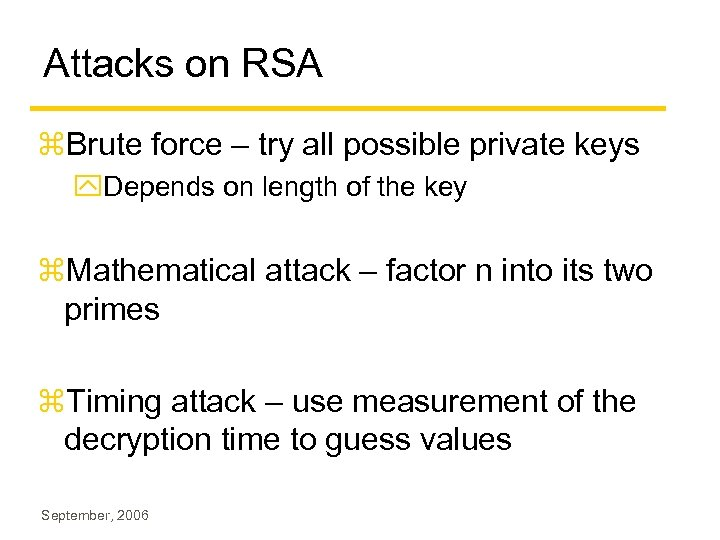Attacks on RSA z. Brute force – try all possible private keys y. Depends on length of the key z. Mathematical attack – factor n into its two primes z. Timing attack – use measurement of the decryption time to guess values September, 2006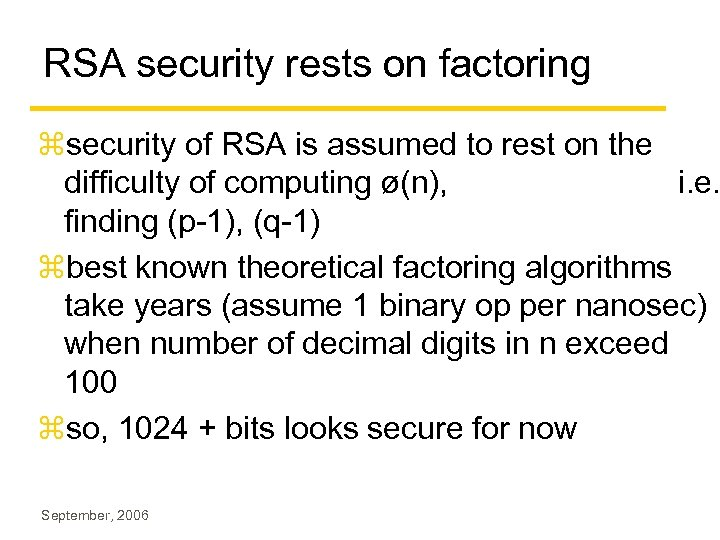RSA security rests on factoring zsecurity of RSA is assumed to rest on the difficulty of computing ø(n), i. e. finding (p-1), (q-1) zbest known theoretical factoring algorithms take years (assume 1 binary op per nanosec) when number of decimal digits in n exceed 100 zso, 1024 + bits looks secure for now September, 2006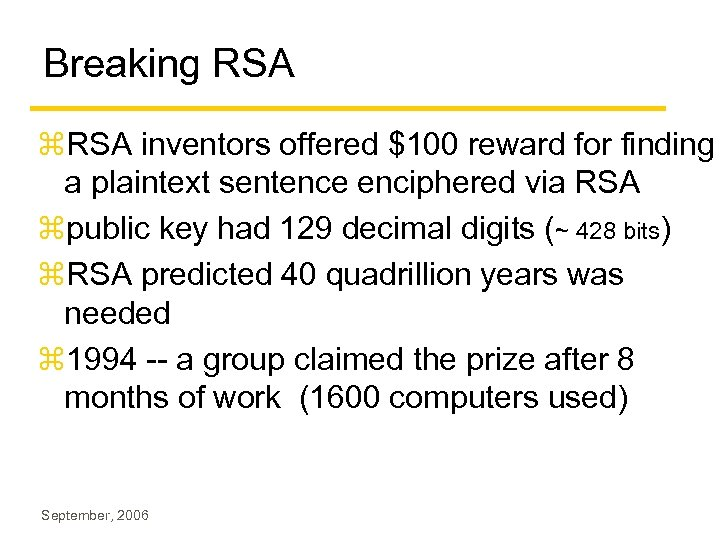Breaking RSA z. RSA inventors offered \$100 reward for finding a plaintext sentence enciphered via RSA zpublic key had 129 decimal digits (~ 428 bits) z. RSA predicted 40 quadrillion years was needed z 1994 -- a group claimed the prize after 8 months of work (1600 computers used) September, 2006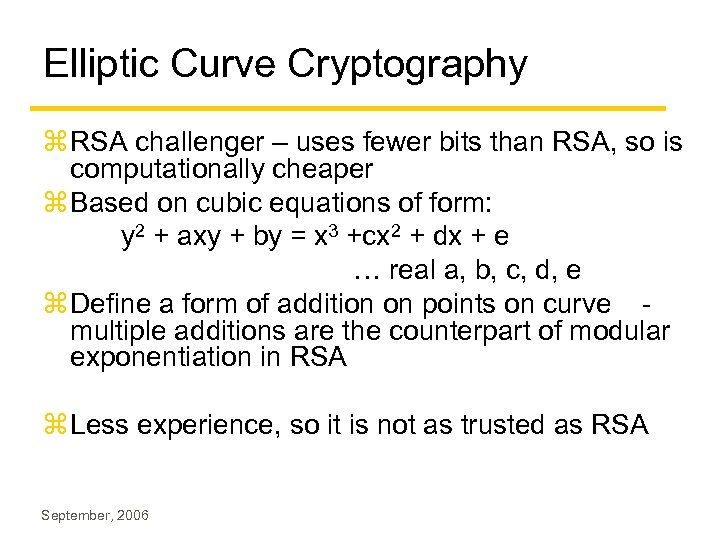Elliptic Curve Cryptography z RSA challenger – uses fewer bits than RSA, so is computationally cheaper z Based on cubic equations of form: y 2 + axy + by = x 3 +cx 2 + dx + e … real a, b, c, d, e z Define a form of addition on points on curve multiple additions are the counterpart of modular exponentiation in RSA z Less experience, so it is not as trusted as RSA September, 2006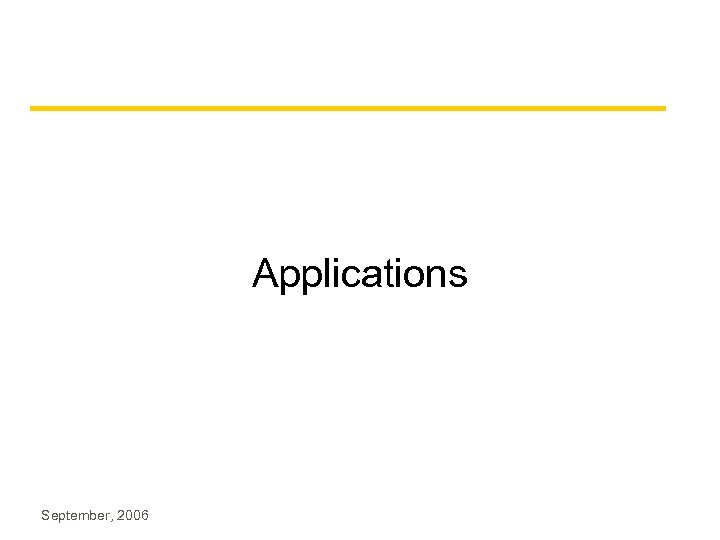Applications September, 2006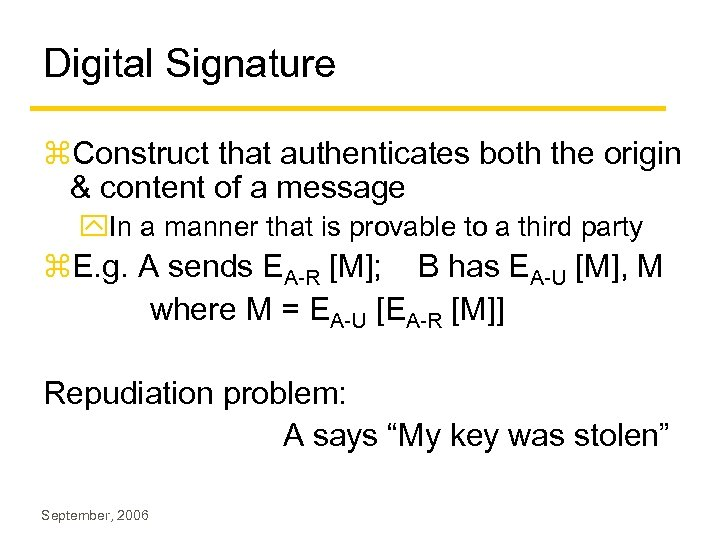Digital Signature z. Construct that authenticates both the origin & content of a message y. In a manner that is provable to a third party z. E. g. A sends EA-R [M]; B has EA-U [M], M where M = EA-U [EA-R [M]] Repudiation problem: A says “My key was stolen” September, 2006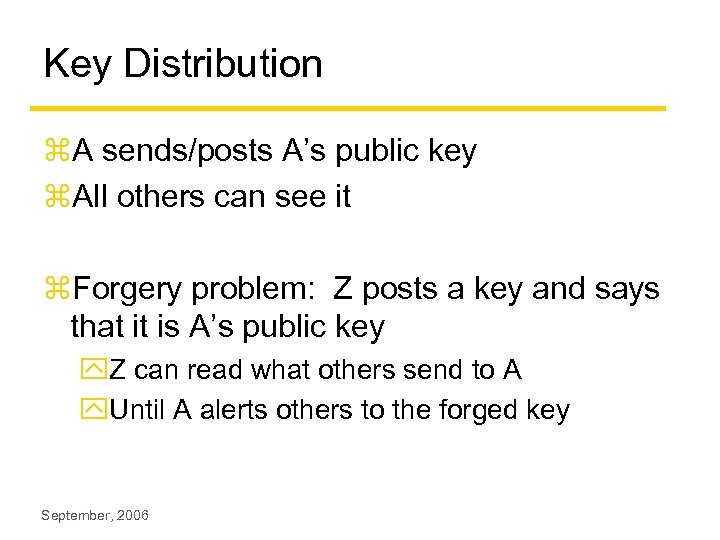Key Distribution z. A sends/posts A’s public key z. All others can see it z. Forgery problem: Z posts a key and says that it is A’s public key y. Z can read what others send to A y. Until A alerts others to the forged key September, 2006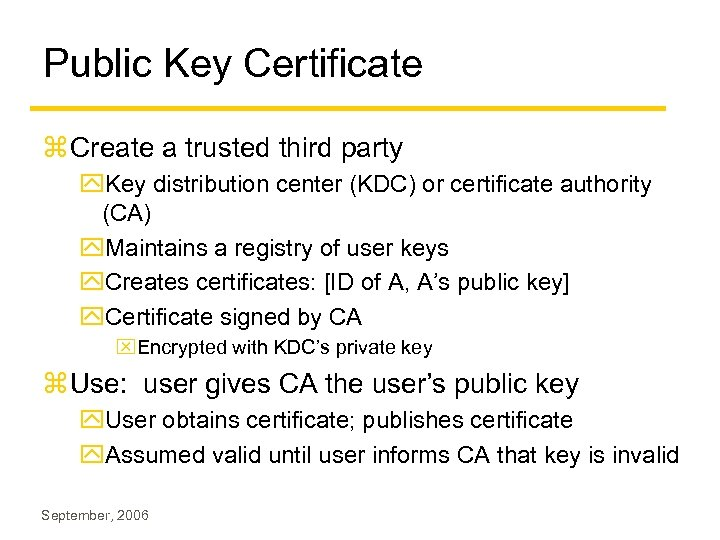Public Key Certificate z Create a trusted third party y. Key distribution center (KDC) or certificate authority (CA) y. Maintains a registry of user keys y. Creates certificates: [ID of A, A’s public key] y. Certificate signed by CA x. Encrypted with KDC’s private key z Use: user gives CA the user’s public key y. User obtains certificate; publishes certificate y. Assumed valid until user informs CA that key is invalid September, 2006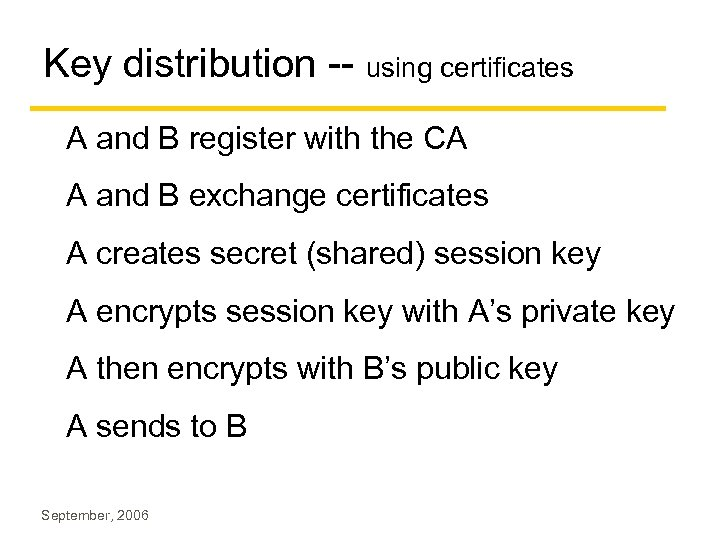Key distribution -- using certificates A and B register with the CA A and B exchange certificates A creates secret (shared) session key A encrypts session key with A’s private key A then encrypts with B’s public key A sends to B September, 2006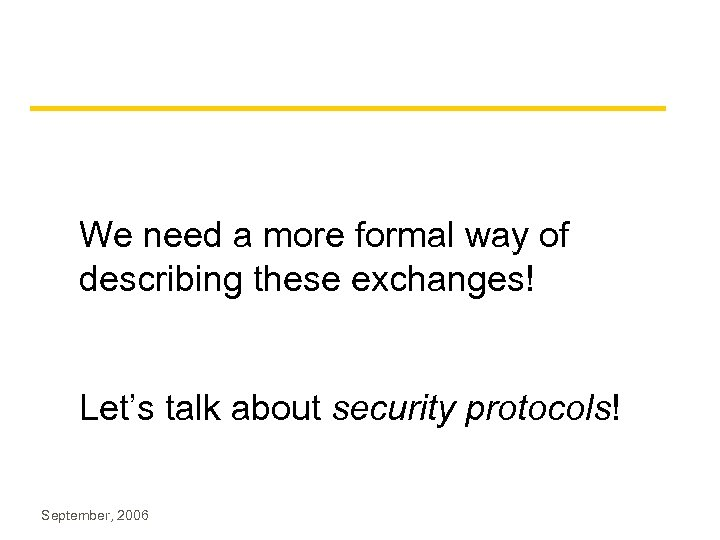We need a more formal way of describing these exchanges! Let’s talk about security protocols! September, 2006Backups September, 2006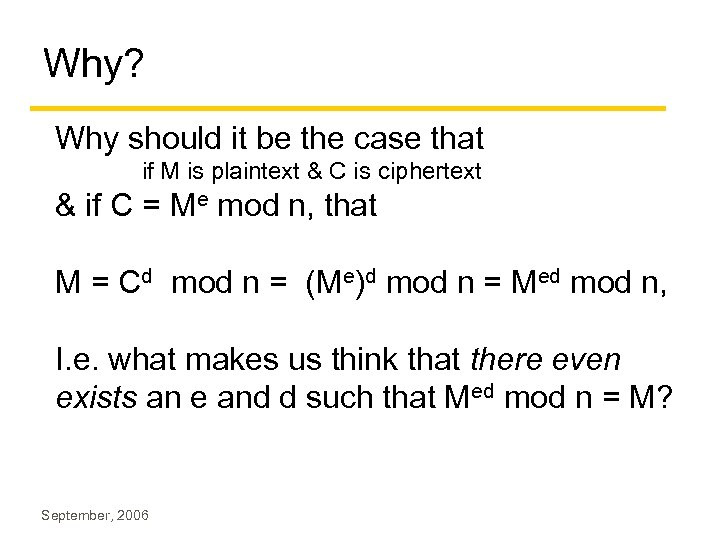Why? Why should it be the case that if M is plaintext & C is ciphertext & if C = Me mod n, that M = Cd mod n = (Me)d mod n = Med mod n, I. e. what makes us think that there even exists an e and d such that Med mod n = M? September, 2006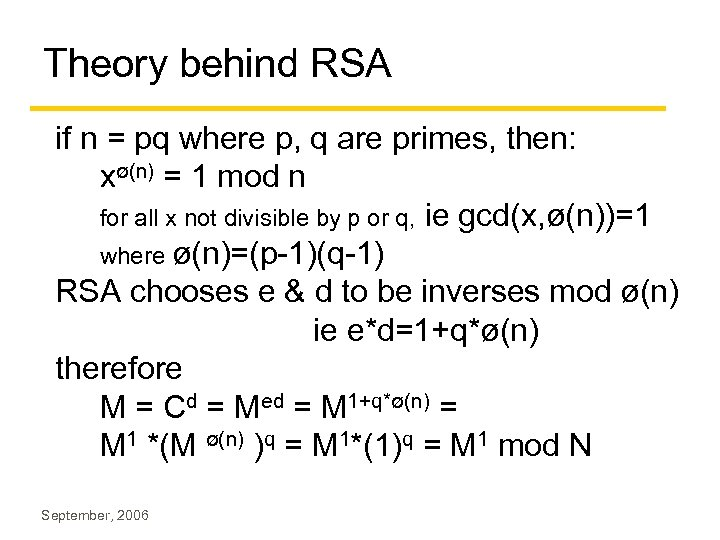Theory behind RSA if n = pq where p, q are primes, then: xø(n) = 1 mod n for all x not divisible by p or q, ie gcd(x, ø(n))=1 where ø(n)=(p-1)(q-1) RSA chooses e & d to be inverses mod ø(n) ie e*d=1+q*ø(n) therefore M = Cd = Med = M 1+q*ø(n) = M 1 *(M ø(n) )q = M 1*(1)q = M 1 mod N September, 2006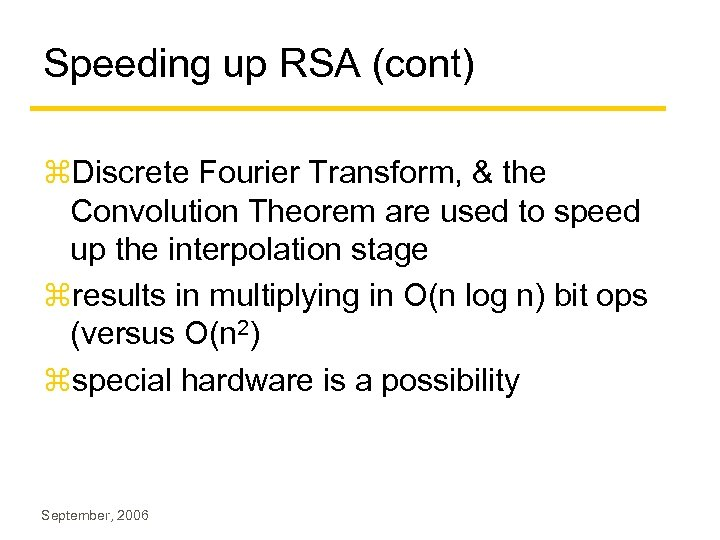Speeding up RSA (cont) z. Discrete Fourier Transform, & the Convolution Theorem are used to speed up the interpolation stage zresults in multiplying in O(n log n) bit ops (versus O(n 2) zspecial hardware is a possibility September, 2006